# CORREL Function

Calculates the correlation coefficient between two variables

## What is the CORREL Function?

The CORREL function is categorized under Excel Statistical functions. It will calculate the correlation coefficient between two variables.

As a financial analyst, the CORREL function is very useful when we want to find the correlation between two variables, e.g., the correlation between a particular stock and a market index.

### Correlation Formula

=CORREL(array1, array2)

The CORREL function uses the following arguments:

1. Array1 (required argument) – This is the set of independent variables. It is a cell range of values.
2. Array2 (required argument) – This is the set of dependent variables. It is the second cell range of values.

The equation for the correlation coefficient is: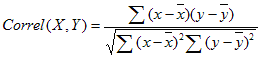Where: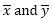are the sample means AVERAGE(array1) and AVERAGE(array2).

So, if the value of r is close to +1, it indicates a strong positive correlation, and if r is close to -1, it shows a strong negative correlation.

### How to use CORREL Function in Excel?

The CORREL function was introduced in Excel 2007 and is available in all subsequent Excel versions. To understand the uses of the function, let’s look at an example:

### Correlation Example

Suppose we are given data about the weekly returns of stock A and percentage of change in a market index (S&P 500):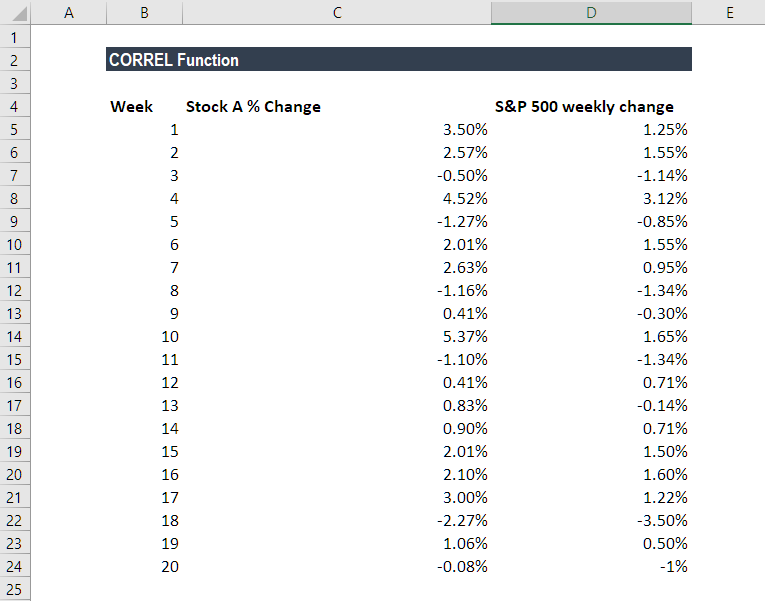The formula used to find the correlation is: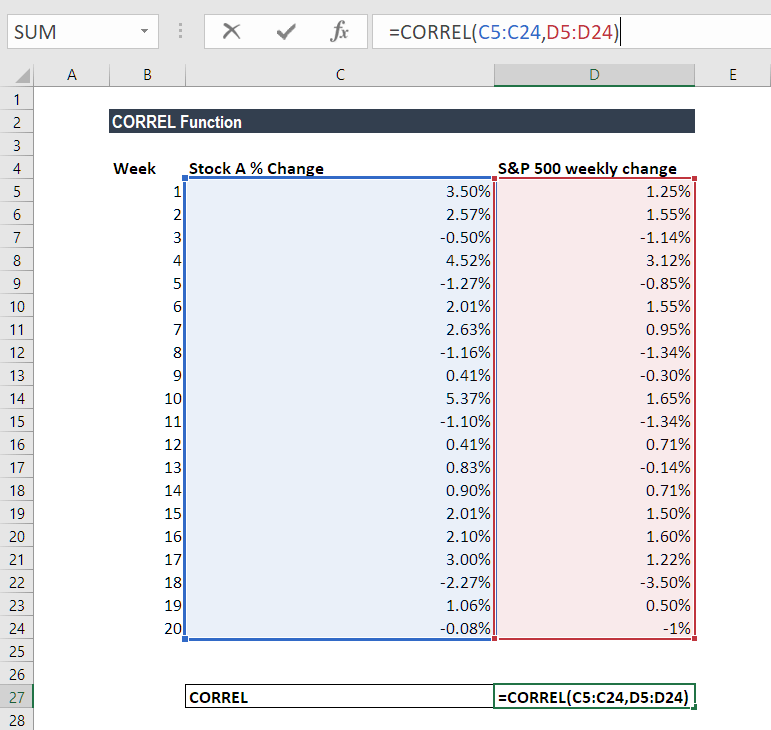We get the result below: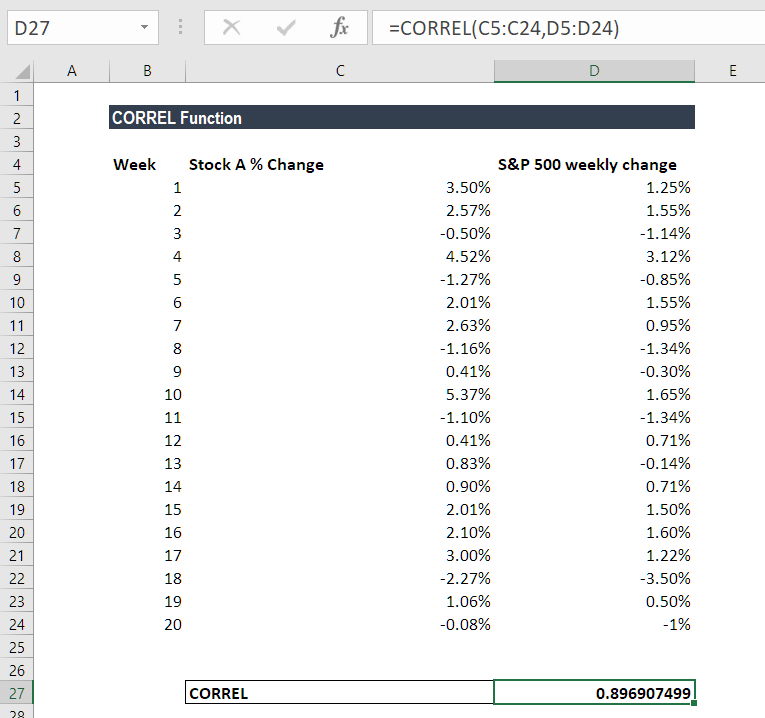The result indicates a strong positive correlation.

### Things to remember about the CORREL Function

1. #N/A error – Occurs if the given arrays are of different lengths. So, if Array1 and Array2 contain different numbers of data points, CORREL will return the #N/A error value.
2. #DIV/0 error – Occurs if either of the given arrays are empty or if the standard deviation of their values equals zero.
3. If an array or reference argument contains text, logical values, or empty cells, the values are ignored; however, cells with the value zero are included.
4. The CORREL function is exactly same as the PEARSON Function, except that, in earlier versions of Excel (earlier than Excel 2003), the PEARSON function may exhibit some rounding errors. Hence, it is advisable to use the CORREL function in earlier versions of Excel. In later versions of Excel, both functions should give the same results.

Thanks for reading CFI’s guide to the Excel CORREL function. By taking the time to learn and master these functions, you’ll significantly speed up your financial analysis. To learn more, check out these additional CFI resources:

• Excel Functions for Finance